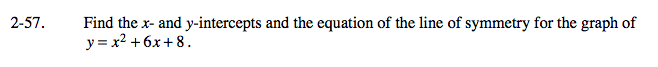### Home > A2C > Chapter 2 > Lesson 2.1.4 > Problem2-57

2-57.Substitute 0 for y and solve to find the x-intercepts.

Substitute 0 for x to find the y-intercept.

Start to sketch the graph by locating the x-intercepts and use the location of the x-intercepts to figure out where the axis of symmetry must be.

The y-intercept is (0, 8), the x-intercepts are (−2, 0) and (−4, 0), and the line of symmetry is x = −3.